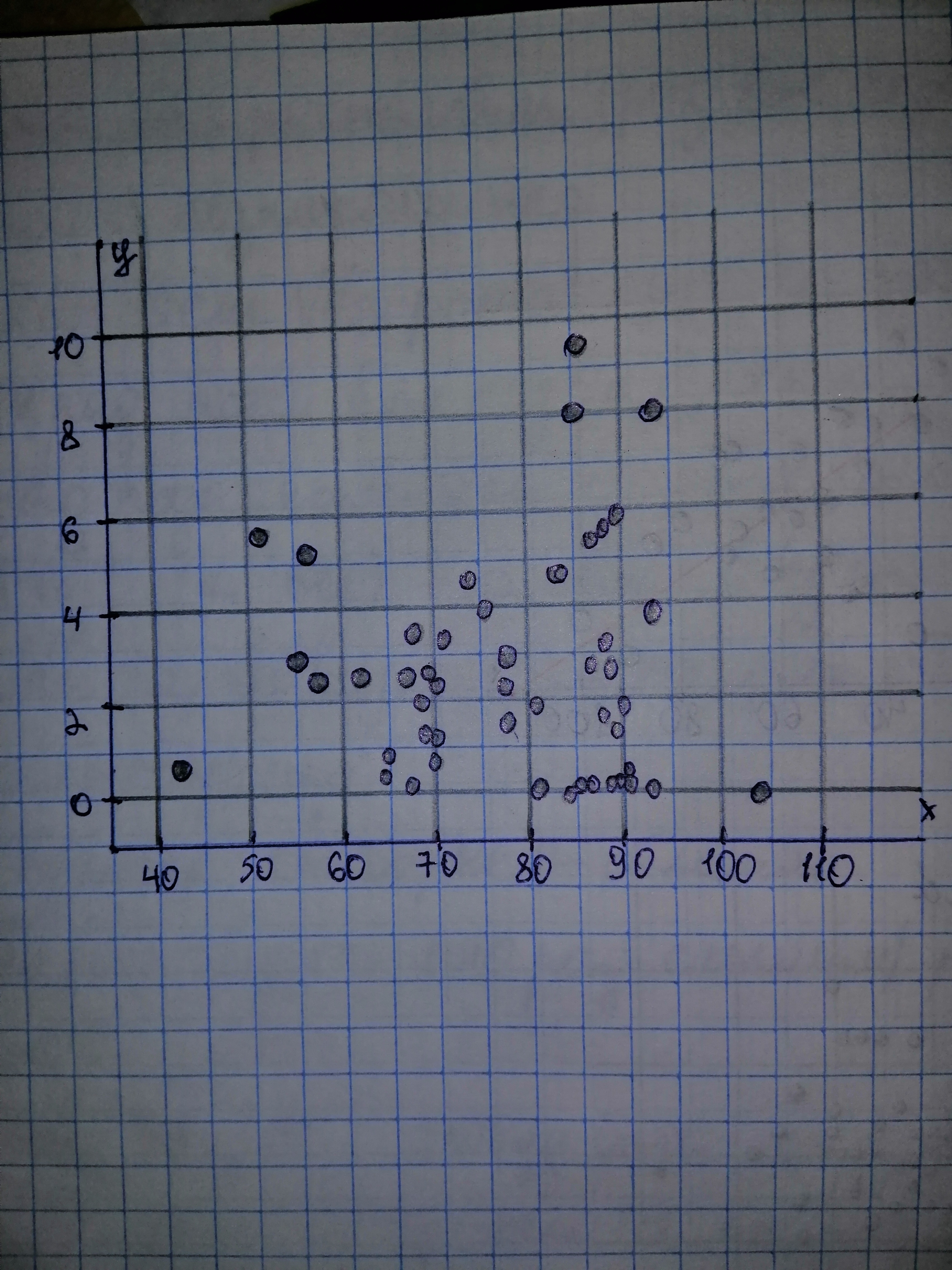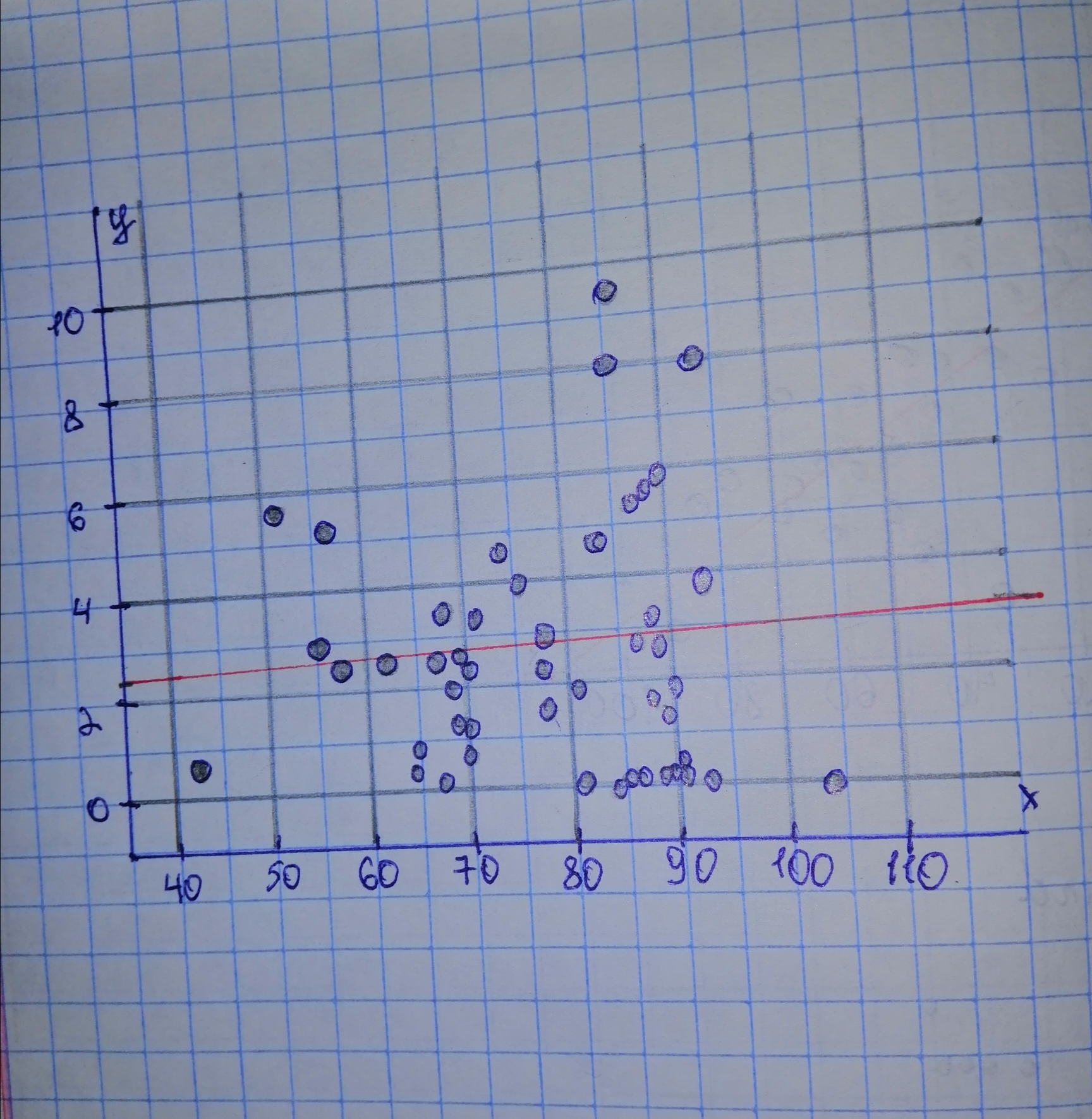# Use the technology of your choice to do the following tasks. The National Oceanic and Atmospheric Administration publishes temperature and precipitatiKye 2020-12-30 Answered
Use the technology of your choice to do the following tasks. The National Oceanic and Atmospheric Administration publishes temperature and precipitation information for cities around the world in Climates of the World. Data on average high temperature (in degrees Fahrenheit) in July and average precipitation (in inches) in July for 48 cities are on the WeissStats CD. For part (d), predict the average July precipitation of a city with an average July temperature of ${83}^{\circ }F$ a) Construct and interpret a scatterplot for the data. b) Decide whether finding a regression line for the data is reasonable. If so, then also do parts (c)-(f). c) Determine and interpret the regression equation. d) Make the indicated predictions. e) Compute and interpret the correlation coefficient. f) Identify potential outliers and influential observations.
You can still ask an expert for help

• Questions are typically answered in as fast as 30 minutes

Solve your problem for the price of one coffee

• Math expert for every subject
• Pay only if we can solve itAamina Herring

Given: a) IMR is on the horizontal axis and LE is on the vertical axis.b) When there is no strong curvature presents in the scatterplot, then it is safe to assume that there is a linear relationship between the variables and thus it is then reasonable to find a regression line. We note that the scatterplot of part (a) does not contain strong curvature and thus is reasonable to find the regression line. c) We determine all necessary sums:

Next, we can determine

The estimate b of the slope
The mean is the sum of all values divided by the number of values:
The estimate a of the intercept
General least-squares equation:d) Let us evalute the regression line in part (c) at $x=83:$